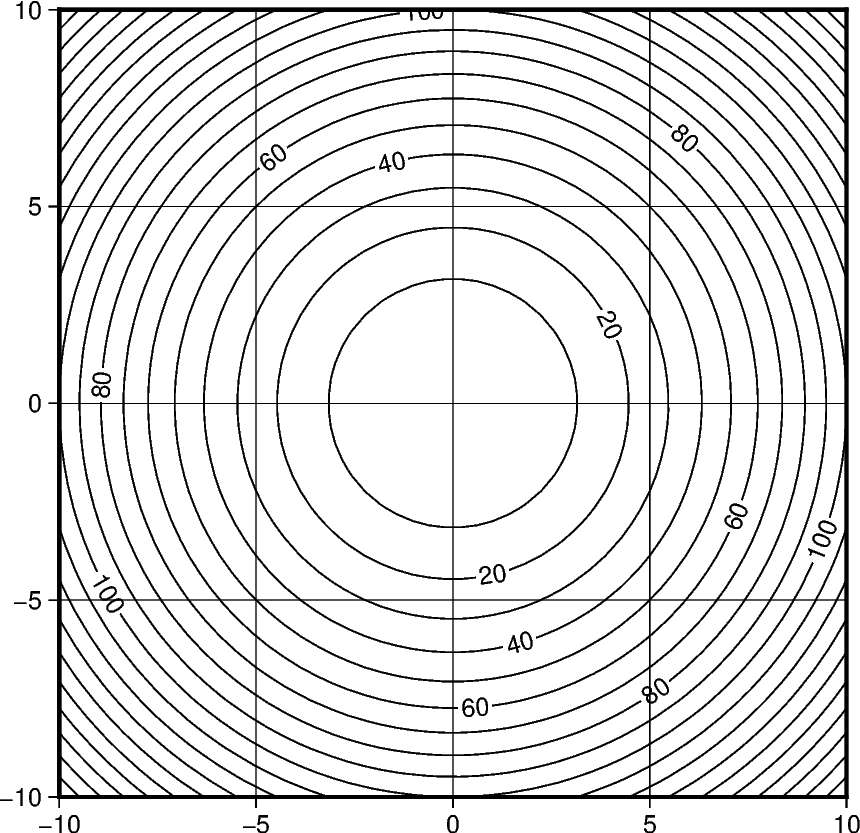# Contours

The `pygmt.Figure.contour` method can plot contour lines from a table of points by direct triangulation. The data for the triangulation can be provided using one of three methods:

1. `x`, `y`, `z` 1d `numpy.ndarray` data columns.

2. `data` 2d `numpy.ndarray` data matrix with 3 columns corresponding to `x`, `y`, `z`.

3. `data` path string to a file containing the `x`, `y`, `z` in a tabular format.

The parameters `levels` and `annotation` set the intervals of the contours and the annotation on the contours respectively.

In this example we supply the data as 1d `numpy.ndarray` with the `x`, `y`, and `z` parameters and draw the contours using a 0.5p pen with contours every 10 `z` values and annotations every 20 `z` values.Out:

```<IPython.core.display.Image object>
```

```import numpy as np
import pygmt

# build the contours underlying data with the function z = x^2 + y^2
X, Y = np.meshgrid(np.linspace(-10, 10, 50), np.linspace(-10, 10, 50))
Z = X ** 2 + Y ** 2
x, y, z = X.flatten(), Y.flatten(), Z.flatten()

fig = pygmt.Figure()
fig.contour(
region=[-10, 10, -10, 10],
projection="X10c/10c",
frame="ag",
pen="0.5p",
# pass the data as 3 1d data columns
x=x,
y=y,
z=z,
# set the contours z values intervals to 10
levels=10,
# set the contours annotation intervals to 20
annotation=20,
)
fig.show()
```

Total running time of the script: ( 0 minutes 0.943 seconds)

Gallery generated by Sphinx-Gallery